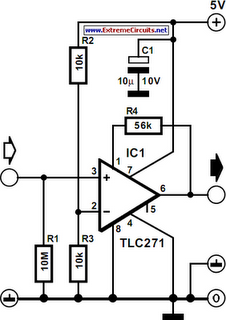# Learning Electronics

Learn to build electronic circuits

# Opamp With Hysteresis

At first glance, the circuit in the diagram does not look out of the ordinary, and yet, it is. This is because it combines two characteristics that are usually assumed to be incompatible: hysteresis and a high input impedance. In a standard op amp circuit, this is, indeed, true, because the creation of hysteresis is normally achieved by positive feedback to the +ve input of the op amp. Unfortunately, the requisite resistance network causes a drastic deterioration of the original high input impedance of the op amp. So, when a high input impedance and hysteresis are wanted, the solution is to obtain the needed positive feedback by coupling the resistor network not to the +ve input but to the offset correction pin.

Circuit diagram:Operational amplifier With Hysteresis Circuit Diagram

When this done, the hysteresis so obtained is calculated from Uh = 1.2/R4Uo, where Uh is the hysteresis voltage and Uo is the output voltage of the op amp, both in volts The value of R4 must be in kΩ. The level of Uo depends, of course, on the load.